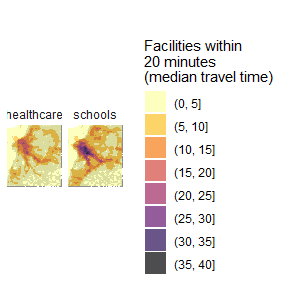# Calculating and visualizing Accessibility

#### 2023-01-27

Abstract

This vignette shows how to calculate and visualize accessibility in R using the r5r package.

# 1. Introduction

Accessibility indicators measure the ease with which opportunities, such as jobs, can be reached by a traveler from a particular location (Levinson and et al. 2020). One of the simplest forms of accessibility metrics is the cumulative-opportunities, which counts all of the opportunities accessible from each location in less than a cutoff time. Using a travel time matrix and information on the number of opportunities available at each location, we can calculate and map accessibility. This vignette shows how to do that in R using the r5r package.

In this reproducible example, we will be using a sample data set for the city of Porto Alegre (Brazil) included in r5r. We can compute accessibility in 5 quick steps:

1. Increase Java memory and load libraries
2. Build routable transport network
3. Calculate Accessibility
4. Map Accessibility

# 2. Build routable transport network with setup_r5()

### Increase Java memory and load libraries

Before we start, we need to increase the memory available to Java and load the packages used in this vignette

options(java.parameters = "-Xmx2G")

library(r5r)
library(sf)
#> Warning: package 'sf' was built under R version 4.1.3
library(data.table)
#> Warning: package 'data.table' was built under R version 4.1.3
library(ggplot2)
library(interp)
#> Warning: package 'interp' was built under R version 4.1.3
library(dplyr)
#> Warning: package 'dplyr' was built under R version 4.1.3

To build a routable transport network with r5r and load it into memory, the user needs to call setup_r5 with the path to the directory where OpenStreetMap and GTFS data are stored.

# system.file returns the directory with example data inside the r5r package
# set data path to directory containing your own data if not using the examples
data_path <- system.file("extdata/poa", package = "r5r")

r5r_core <- setup_r5(data_path)

# 3. Calculate Accessibility

In this example, we will be calculating the number of schools and public healthcare facilities accessible by public transport within a travel time of up to 20 minutes. The sample data provided contains information on the spatial distribution of schools in Porto Alegre in the points$schools column, and healthcare facilities in the points$healthcare column.

With the code below we compute the number of schools and healthcare accessible considering median of multiple travel time estimates departing every minute over a 60-minute time window, between 2pm and 3pm. The accessibility() function can calculate access to multiple opportunities in a single call, which is much more efficient and convenient than producing a travel time matrix of the study area and manually computing accessibility.

# read all points in the city

# routing inputs
mode <- c("WALK", "TRANSIT")
max_walk_time <- 30 # in minutes
travel_time_cutoff <- 21 # in minutes
departure_datetime <- as.POSIXct("13-05-2019 14:00:00",
format = "%d-%m-%Y %H:%M:%S")

time_window <- 60 # in minutes
percentiles <- 50

# calculate travel time matrix
access <- accessibility(r5r_core,
origins = points,
destinations = points,
mode = mode,
opportunities_colnames = c("schools", "healthcare"),
decay_function = "step",
cutoffs = travel_time_cutoff,
departure_datetime = departure_datetime,
max_walk_time = max_walk_time,
time_window = time_window,
percentiles = percentiles,
progress = FALSE)

#>                 id opportunity percentile cutoff accessibility
#> 1: 89a901291abffff     schools         50     21             3
#> 2: 89a901291abffff  healthcare         50     21             6
#> 3: 89a9012a3cfffff     schools         50     21             0
#> 4: 89a9012a3cfffff  healthcare         50     21             0
#> 5: 89a901295b7ffff     schools         50     21             6
#> 6: 89a901295b7ffff  healthcare         50     21             4

# 4. Map Accessibility

The final step is mapping the accessibility results calculated earlier. The code below demonstrates how to do that, with some extra steps to produce a prettier map by doing a spatial interpolation of accessibility estimates.

# interpolate estimates to get spatially smooth result
access_schools <- access %>%
filter(opportunity == "schools") %>%
inner_join(points, by='id') %>%
with(interp::interp(lon, lat, accessibility)) %>%
with(cbind(acc=as.vector(z),  # Column-major order
x=rep(x, times=length(y)),
y=rep(y, each=length(x)))) %>% as.data.frame() %>% na.omit() %>%
mutate(opportunity = "schools")

access_health <- access %>%
filter(opportunity == "healthcare") %>%
inner_join(points, by='id') %>%
with(interp::interp(lon, lat, accessibility)) %>%
with(cbind(acc=as.vector(z),  # Column-major order
x=rep(x, times=length(y)),
y=rep(y, each=length(x)))) %>% as.data.frame() %>% na.omit() %>%
mutate(opportunity = "healthcare")

access.interp <- rbind(access_schools, access_health)

# find results' bounding box to crop the map
bb_x <- c(min(access.interp$x), max(access.interp$x))
bb_y <- c(min(access.interp$y), max(access.interp$y))

# extract OSM network, to plot over map
street_net <- street_network_to_sf(r5r_core)

# plot
ggplot(na.omit(access.interp)) +
geom_sf(data = street_net\$edges, color = "gray55", size=0.01, alpha = 0.7) +
geom_contour_filled(aes(x=x, y=y, z=acc), alpha=.7) +
scale_fill_viridis_d(direction = -1, option = 'B') +
scale_x_continuous(expand=c(0,0)) +
scale_y_continuous(expand=c(0,0)) +
coord_sf(xlim = bb_x, ylim = bb_y, datum = NA) +
labs(fill = "Facilities within\n20 minutes\n(median travel time)") +
theme_minimal() +
theme(axis.title = element_blank()) +
facet_wrap(~opportunity)### Cleaning up after usage

r5r objects are still allocated to any amount of memory previously set after they are done with their calculations. In order to remove an existing r5r object and reallocate the memory it had been using, we use the stop_r5 function followed by a call to Java’s garbage collector, as follows:

r5r::stop_r5(r5r_core)
rJava::.jgc(R.gc = TRUE)

If you have any suggestions or want to report an error, please visit the package GitHub page.